﻿ 一类p-Laplace方程解的存在性问题 Existence of Solution for One Class of p-Laplacian Problem

Pure Mathematics
Vol. 09  No. 03 ( 2019 ), Article ID: 30136 , 8 pages
10.12677/PM.2019.93041

Existence of Solution for One Class of p-Laplacian Problem

Lei Li

Department of Mathematics and Statistics, Guangxi Normal University, Guilin Guangxi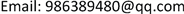Received: Apr. 16th, 2019; accepted: Apr. 27th, 2019; published: May 9th, 2019ABSTRACT

In this paper, we study the existence of weak solutions for the Dirichlet problems for one class of nonlinear p-Laplacian equations. Our proof combines the presence of sub and supersolution with the pseudomonotone operators theory.

Keywords:Sub and Supersolution, Pseudomonotone Operators Theory, p-Laplacian Equations1. 引言

$\left\{\begin{array}{l}-a\left(\int {|u|}^{q}\right)div\left({|\nabla u|}^{p-2}\nabla u\right)={h}_{1}\left(x,u\right)f\left(\int {|u|}^{m}\right)+{h}_{2}\left(x,u\right)g\left(\int {|u|}^{r}\right),\text{\hspace{0.17em}}\text{\hspace{0.17em}}\text{\hspace{0.17em}}\text{\hspace{0.17em}}x\in \Omega ,\\ u=0,x\in \partial \Omega .\end{array}$ (1)

(H1) $\Omega \subset {R}^{N}$，是一个有界的区域且边界光滑。

(H2) $q,m,r\in \left[1,+\infty \right)$

(H3)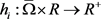是连续的函数。

(H4) $a,f,g:\left[0,+\infty \right)\to \left(0,+\infty \right)$ 是连续的函数且 $f,g\in {L}^{\infty }\left(\left[0,+\infty \right)\right)$

(H5) $\underset{t\in \left[0,+\infty \right)}{\mathrm{inf}}a\left(t\right),\underset{t\in \left[0,+\infty \right)}{\mathrm{inf}}f\left(t\right),\underset{t\in \left[0,+\infty \right)}{\mathrm{inf}}g\left(t\right)\ge {a}_{0}>0$

$\left\{\begin{array}{l}-a\left(\int {|u|}^{q}\right)\Delta u={h}_{1}\left(x,u\right)f\left(\int {|u|}^{m}\right)+{h}_{2}\left(x,u\right)g\left(\int {|u|}^{r}\right),\text{\hspace{0.17em}}\text{\hspace{0.17em}}\text{\hspace{0.17em}}\text{\hspace{0.17em}}x\in \Omega ,\\ u=0,x\in \partial \Omega .\end{array}$ (2)

$\underset{_}{u}\left(x\right)\le 0\le \stackrel{¯}{u}\left(x\right),x\in \partial \Omega ,$(3)

$-{\Delta }_{p}\stackrel{¯}{u}\ge \frac{1}{{a}_{0}}\left({h}_{1}\left(x,\stackrel{¯}{u}\right){‖f‖}_{\infty }+{h}_{2}\left(x,\stackrel{¯}{u}\right){‖g‖}_{\infty }\right),\text{\hspace{0.17em}}\text{\hspace{0.17em}}x\in \Omega .$ (4)

$-{\Delta }_{p}\underset{_}{u}\le \frac{{a}_{0}}{\Gamma }\left({h}_{1}\left(x,\underset{_}{u}\right)+{h}_{2}\left(x,\underset{_}{u}\right)\right),\text{\hspace{0.17em}}\text{\hspace{0.17em}}x\in \Omega .$ (5)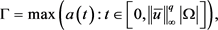(6)

$a\left(\int {|u|}^{q}\right)\int {|\nabla u|}^{p-2}\nabla u\nabla \varphi =f\left(\int {|u|}^{m}\right)\int {h}_{1}\left(x,u\right)\varphi +g\left(\int {|u|}^{r}\right)\int {h}_{2}\left(x,u\right)\varphi$ (7)

2. 预备知识

a) 是有界算子，如果 $\forall u\in X$$\left\{Bu\right\}$${X}^{*}$ 中的有界凸集。

b) 是强制算子，如果满足

$\underset{‖u‖\to \infty }{\mathrm{lim}}\frac{〈Bu,u〉}{‖u‖}=\infty .$ (8)

c) 是伪单调算子，如果满足 ${u}_{n}\to u$$\sigma \left(X,{X}^{*}\right)$ 中，且

$\underset{n\to \infty }{\mathrm{lim}}\mathrm{sup}〈B{u}_{n},{u}_{n}-u〉\le 0,$ (9)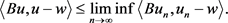(10)

$\left({|\xi |}^{p-2}\xi -{|\eta |}^{p-2}\eta \right)\left(\xi -\eta \right)\ge {2}^{2-p}{|\xi -\eta |}^{p}.$ (11)

3. 定理1的证明

$\zeta \left(x,t\right)=\left\{\begin{array}{ll}\underset{_}{u}\left(x\right),\hfill & t\le \underset{_}{u}\left(x\right),\hfill \\ t,\hfill & \underset{_}{u}\left(x\right)\le t\le \stackrel{¯}{u}\left(x\right),\hfill \\ \stackrel{¯}{u}\left(x\right),\hfill & t\ge \stackrel{¯}{u}\left(x\right).\hfill \end{array}$$\gamma \left(x,t\right)=-{\left(\underset{_}{u}-t\right)}_{+}^{l}+{\left(t-\stackrel{¯}{u}\right)}_{+}^{l},$ (12)

$\left\{\begin{array}{l}-a\left(\int {|u|}^{q}\right){\Delta }_{p}u=H\left(x,u,f\left(\int {|\zeta \left(x,u\right)|}^{m}\right),g\left(\int {|\zeta \left(x,u\right)|}^{r}\right)\right),\text{\hspace{0.17em}}\text{\hspace{0.17em}}\text{\hspace{0.17em}}\text{\hspace{0.17em}}x\in \Omega ,\\ u=0,x\in \partial \Omega .\end{array}$ (13)

$u<\underset{_}{u}$ 时： $|H\left(x,u,f,g\right)|={\stackrel{^}{h}}_{1}f+{\stackrel{^}{h}}_{2}g+{\left(\underset{_}{u}-u\right)}_{+}^{l}>0$，此时，对方程(13)用最值原理易得： $\underset{_}{u}>u>0$，因此：

$\begin{array}{c}|H\left(x,u,f,g\right)|=|{\stackrel{^}{h}}_{1}f+{\stackrel{^}{h}}_{2}g+{\left(\underset{_}{u}-u\right)}_{+}^{l}|\\ \le |{\stackrel{^}{h}}_{1}f|+|{\stackrel{^}{h}}_{2}g|+|{\left(\underset{_}{u}-u\right)}_{+}^{l}|\\ \le |{\stackrel{^}{h}}_{1}f|+|{\stackrel{^}{h}}_{2}g|+{|\underset{_}{u}|}^{l}.\end{array}$ (14)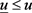时：

$|H\left(x,u,f,g\right)|\le |{\stackrel{^}{h}}_{1}f+{\stackrel{^}{h}}_{2}g|.$ (15)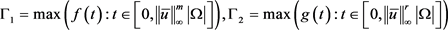，利用上述条件以及Minkowski不等式我们有：

$\begin{array}{c}{\left(\int {|H\left(x,u,f,g\right)|}^{{p}^{*}}\right)}^{\frac{1}{{p}^{*}}}\le {\left(\int {|{\stackrel{^}{h}}_{1}f|}^{{p}^{*}}\right)}^{\frac{1}{{p}^{*}}}+{\left(\int {|{\stackrel{^}{h}}_{2}g|}^{{p}^{*}}\right)}^{\frac{1}{{p}^{*}}}+{\left(\int {|\underset{_}{u}|}^{l{p}^{*}}\right)}^{\frac{1}{{p}^{*}}}\\ \le {\Gamma }_{1}{\left(\int {|{\stackrel{^}{h}}_{1}|}^{{p}^{*}}\right)}^{\frac{1}{{p}^{*}}}+{\Gamma }_{2}{\left(\int {|{\stackrel{^}{h}}_{2}|}^{{p}^{*}}\right)}^{\frac{1}{{p}^{*}}}+{\left(\int {|\underset{_}{u}|}^{l{p}^{*}}\right)}^{\frac{1}{{p}^{*}}}\\ \le {\Gamma }_{1}{M}_{1}{|\Omega |}^{\frac{1}{{p}^{*}}}+{\Gamma }_{2}{M}_{2}{|\Omega |}^{\frac{1}{{p}^{*}}}+{\left(\int {|\underset{_}{u}|}^{l{p}^{*}}\right)}^{\frac{1}{{p}^{*}}}\\ <\infty \end{array}$

$〈B\left(u\right),v〉=a\left(\int {|\zeta \left(x,u\right)|}^{q}\right)\int {|\nabla u|}^{p-2}\nabla u\nabla v-\int H\left(x,u,f\left(\int {|\zeta \left(x,u\right)|}^{m}\right),g\left(\int {|\zeta \left(x,u\right)|}^{r}\right)\right)v,$ (16)

$\begin{array}{c}〈B\left(u\right),v〉=a\left(\int {|\zeta \left(x,u\right)|}^{q}\right)\int {|\nabla u|}^{p-2}\nabla u\nabla v-\int H\left(x,u,f\left(\int {|\zeta \left(x,u\right)|}^{m}\right),g\left(\int {|\zeta \left(x,u\right)|}^{r}\right)\right)v\\ \le a\left(\int {|\zeta \left(x,u\right)|}^{q}\right)\int {|\nabla u|}^{p-1}|\nabla v|+\int |H\left(x,u,f\left(\int {|\zeta \left(x,u\right)|}^{m}\right),g\left(\int {|\zeta \left(x,u\right)|}^{r}\right)\right)||v|\\ \le \Gamma {\left(\int {|\nabla u|}^{p}\right)}^{\frac{1}{{p}^{*}}}{\left(\int {|\nabla v|}^{p}\right)}^{\frac{1}{p}}+{\left(\int {|H\left(x,u,f\left(\int {|\zeta \left(x,u\right)|}^{m}\right),g\left(\int {|\zeta \left(x,u\right)|}^{r}\right)\right)|}^{{p}^{*}}\right)}^{\frac{1}{{p}^{*}}}{\left(\int {|v|}^{p}\right)}^{\frac{1}{p}}\\ \le C{‖v‖}_{{W}_{0}^{1,p}\left(\Omega \right)},\end{array}$ (17)

$\begin{array}{c}\frac{〈B\left(u\right),v〉}{‖u‖}=\frac{a\left(\int {|\zeta \left(x,u\right)|}^{q}\right)\int {|\nabla u|}^{p}-\int H\left(x,u,f\left(\int {|\zeta \left(x,u\right)|}^{m}\right),g\left(\int {|\zeta \left(x,u\right)|}^{r}\right)u\right)}{‖u‖}\\ \ge {a}_{0}{\left(\int {|\nabla u|}^{p}\right)}^{1-\frac{1}{p}}-\frac{{\left(\int {|H\left(x,u,f\left(\int {|\zeta \left(x,u\right)|}^{m}\right),g\left(\int {|\zeta \left(x,u\right)|}^{r}\right)\right)|}^{{p}^{*}}\right)}^{\frac{1}{{p}^{*}}}{\left(\int {|u|}^{p}\right)}^{\frac{1}{p}}}{‖u‖},\end{array}$

$\left(‖u‖={\left(\int {|\nabla u|}^{p}\right)}^{\frac{1}{p}}\right),$

$‖u‖\to \infty$ 时，则

$\frac{〈B\left(u\right),v〉}{‖u‖}\to \infty ,$

$\underset{n\to \infty }{\mathrm{lim}}\mathrm{sup}〈B\left({u}_{n}\right),{u}_{n}-u〉\le 0,$ (18)

$\underset{n\to \infty }{\mathrm{lim}}\mathrm{inf}〈B\left({u}_{n}\right),{u}_{n}-v〉\ge 〈B\left(u\right),u-v〉,\forall v\in {W}_{0}^{1,p}\left(\Omega \right).$ (19)$\underset{n\to \infty }{\mathrm{lim}}\mathrm{sup}〈B\left({u}_{n}\right),{u}_{n}-u〉\le 0,$

$\underset{n\to \infty }{\mathrm{lim}}\mathrm{sup}\int {|\nabla {u}_{n}|}^{p-2}\nabla {u}_{n}\left(\nabla {u}_{n}-\nabla u\right)\le 0.$ (20)

$\underset{n\to \infty }{\mathrm{lim}}〈B\left(u\right),{u}_{n}-u〉=0,$

$\underset{n\to \infty }{\mathrm{lim}}\int {|\nabla u|}^{p-2}\nabla u\left(\nabla {u}_{n}-\nabla u\right)=0.$ (21)

$0\ge \underset{n\to \infty }{\mathrm{lim}}\int \left({|\nabla {u}_{n}|}^{p-2}\nabla {u}_{n}-{|\nabla u|}^{p-2}\nabla u\right)\left(\nabla {u}_{n}-\nabla u\right)\ge \underset{n\to \infty }{\mathrm{lim}}\int {2}^{2-p}{|\nabla {u}_{n}-\nabla u|}^{p}\ge 0,$(22)

$\int {|\nabla {u}_{n}-\nabla u|}^{p}\to 0,$ (23)

$\underset{n\to \infty }{\mathrm{lim}}〈B\left({u}_{n}\right)-B\left(u\right),{u}_{n}-u〉=0,$ (24)

$\begin{array}{c}\underset{n\to \infty }{\mathrm{lim}}\mathrm{inf}〈B\left({u}_{n}\right),{u}_{n}-v〉\ge \underset{n\to \infty }{\mathrm{lim}}\mathrm{inf}〈B\left({u}_{n}\right),{u}_{n}〉-\underset{n\to \infty }{\mathrm{lim}}\mathrm{sup}〈B\left({u}_{n}\right),v〉\\ =\underset{n\to \infty }{\mathrm{lim}}\mathrm{inf}〈B\left({u}_{n}\right),{u}_{n}-u〉+\underset{n\to \infty }{\mathrm{lim}}〈B\left({u}_{n}\right),u〉-〈B\left(u\right),v〉\\ =\underset{n\to \infty }{\mathrm{lim}}〈B\left(u\right),{u}_{n}-u〉+〈B\left(u\right),u〉-〈B\left(u\right),v〉\\ =〈B\left(u\right),u〉-〈B\left(u\right),v〉\\ =〈B\left(u\right),u-v〉.\end{array}$ (25)

$\begin{array}{l}a\left(\int {|u|}^{q}\right)\int {|\nabla u|}^{p-2}\nabla u\nabla {\left(u-\stackrel{¯}{u}\right)}_{+}\\ =\int H\left(x,u,f\left(\int {|\zeta \left(x,u\right)|}^{m}\right),g\left(\int {|\zeta \left(x,u\right)|}^{r}\right)\right){\left(u-\stackrel{¯}{u}\right)}_{+},\end{array}$

$\begin{array}{l}a\left(\int {|u|}^{q}\right)\int {|\nabla u|}^{p-2}\nabla u\nabla {\left(u-\stackrel{¯}{u}\right)}_{+}\\ =f\left(\int {|\zeta \left(x,u\right)|}^{m}\right)\int {h}_{1}\left(x,u\right){\left(u-\stackrel{¯}{u}\right)}_{+}+g\left(\int {|\zeta \left(x,u\right)|}^{r}\right)\int {h}_{2}\left(x,u\right){\left(u-\stackrel{¯}{u}\right)}_{+}-\int \gamma \left(x,u\right){\left(u-\stackrel{¯}{u}\right)}_{+},\end{array}$

$\begin{array}{l}\int {|\nabla u|}^{p-2}\nabla u\nabla {\left(u-\stackrel{¯}{u}\right)}_{+}\\ \le \frac{1}{{a}_{0}}{‖f‖}_{\infty }\int {h}_{1}\left(x,\stackrel{¯}{u}\right){\left(u-\stackrel{¯}{u}\right)}_{+}+\frac{1}{{a}_{0}}{‖g‖}_{\infty }\int {h}_{2}\left(x,\stackrel{¯}{u}\right){\left(u-\stackrel{¯}{u}\right)}_{+}-\frac{1}{a\left(\int {|u|}^{q}\right)}\int {\left(u-\stackrel{¯}{u}\right)}_{+}^{l+1}.\end{array}$

$\int {|\nabla u|}^{p-2}\nabla u\nabla {\left(u-\stackrel{¯}{u}\right)}_{+}\le \int {|\nabla \stackrel{¯}{u}|}^{p-2}\nabla \stackrel{¯}{u}\nabla {\left(u-\stackrel{¯}{u}\right)}_{+}-a\left(\int {|u|}^{q}\right)\int {\left(u-\stackrel{¯}{u}\right)}_{+}^{l+1},$

$\int {|\nabla u|}^{p-2}\nabla u\nabla {\left(u-\stackrel{¯}{u}\right)}_{+}-\int {|\nabla \stackrel{¯}{u}|}^{p-2}\nabla \stackrel{¯}{u}\nabla {\left(u-\stackrel{¯}{u}\right)}_{+}\le -a\left(\int {|u|}^{q}\right)\int {\left(u-\stackrel{¯}{u}\right)}_{+}^{l+1}\le 0,$

$a\left(\int {|u|}^{q}\right)\int {|\nabla u|}^{p-2}\nabla u\nabla {\left(\underset{_}{u}-u\right)}_{+}=\int H\left(x,u,f\left(\int {|\zeta \left(x,u\right)|}^{m}\right),g\left(\int {|\zeta \left(x,u\right)|}^{r}\right)\right){\left(\underset{_}{u}-u\right)}_{+},$

$\begin{array}{l}a\left(\int {|u|}^{q}\right)\int {|\nabla u|}^{p-2}\nabla u\nabla {\left(\underset{_}{u}-u\right)}_{+}\\ =f\left(\int {|\zeta \left(x,u\right)|}^{m}\right)\int {h}_{1}\left(x,u\right){\left(\underset{_}{u}-u\right)}_{+}+g\left(\int {|\zeta \left(x,u\right)|}^{r}\right)\int {h}_{2}\left(x,u\right){\left(\underset{_}{u}-u\right)}_{+}-\int \gamma \left(x,u\right){\left(\underset{_}{u}-u\right)}_{+}.\end{array}$$\begin{array}{l}\int {|\nabla u|}^{p-2}\nabla u\nabla {\left(\underset{_}{u}-u\right)}_{+}\\ \ge \frac{{a}_{0}}{\Gamma }\int {h}_{1}\left(x,\underset{_}{u}\right)\left(\underset{_}{u}-u\right)+\frac{{a}_{0}}{\Gamma }\int {h}_{1}\left(x,\underset{_}{u}\right)\left(\underset{_}{u}-u\right)+\frac{1}{\Gamma }\int {\left(\underset{_}{u}-u\right)}_{+}^{l+1}\\ \ge \int {|\nabla \underset{_}{u}|}^{p-2}\nabla \underset{_}{u}\nabla {\left(\underset{_}{u}-u\right)}_{+}+\frac{1}{\Gamma }\int {\left(\underset{_}{u}-u\right)}_{+}^{l+1},\end{array}$ (26)

$\int {|\nabla \underset{_}{u}|}^{p-2}\nabla u\nabla {\left(\underset{_}{u}-u\right)}_{+}-\int {|\nabla u|}^{p-2}\nabla u\nabla {\left(\underset{_}{u}-u\right)}_{+}\le -\frac{1}{\Gamma }\int {\left(\underset{_}{u}-u\right)}_{+}^{l+1}\le 0,$

$左边\ge \int {2}^{2-p}{|\nabla {\left(\underset{_}{u}-u\right)}_{+}|}^{p}\ge 0,$

Existence of Solution for One Class of p-Laplacian Problem[J]. 理论数学, 2019, 09(03): 308-315. https://doi.org/10.12677/PM.2019.93041

1. 1. Alves, C.O. and Covei, D.P. (2015) Existence of Solution for a Class of Nonlocal Elliptic Problem via Sub-Supersolution Method. Nonlinear Analysis Real World Applications, 23, 1-8.
https://doi.org/10.1016/j.nonrwa.2014.11.003

2. 2. Zeidler, E. (1991) Nonlinear Functional Analysis and Its Applications, Part I: Fixed-Point Theorems. Acta Applicandae Mathematica, 24, 312-314.

3. 3. 李开泰, 马逸尘. 数学物理方程Hilbert空间方法[M]. 北京: 科学出版社, 2008.

4. 4. Troianiello, G.M. (2010) Elliptic Differential Equations and Obstacle Problems. University Series in Mathematics. Springer US, New York.

5. 5. 张恭庆, 林源渠. 泛函分析讲义[M]. 北京: 北京大学出版社, 1987.

6. 6. 孙炯, 王万义, 郝建文. 泛函分析[M]. 北京: 高等教育出版社, 2010.

7. 7. Peral, I. (2010) Multiplicity of Solutions for the p-Laplacian. http://matematicas.uam.es/~ireneo.peral/ICTP.pdf

8. 8. 王明新. 索伯列夫空间[M]. 北京: 高等教育出版社, 2013.